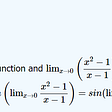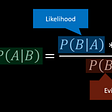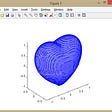# What is the difference between Probability and Likelihood?

Let us understand these two statistical terms in an easy way!!

For me, visualizations are easy to understand so I will try to put the difference between Probability and Likelihood using simple visualization. The following explanation applies to all the continuous distributions but for now, let’s start by looking at the probability with respect to a normal distribution.

Example:

Let’s imagine that the below distribution is of 3 years old kids height. On the low end, we have 2.4 feet and on the high end, we have 3.4 feet. It has an average of 2.9 feet in height and a standard deviation of 0.5.

The probability that we will weigh a randomly selected height between 2.9 and 3.0 feet is the area under the curve between 2.9 and 3.0 feet.

In this case, the area under the curve = 0.21, which means there is a 21% chance that a randomly selected 3-year old kid would have a height between 2.9 and 3.0 feet.

Statistically, we say this with the following notation,

Prob(height between 2.9 feet and 3.0 feet | mean = 2.9 and standard deviation =0.5) = 0.21 i.e., 21% is the probability of height of a 3-yearold kid is between 2.9 feet and 3.0 feet given that the mean of the distribution is 2.9 and the standard deviation is 0.5.

The highlighted part of the below equation can be changed based on our requirement of the study.

Prob(height between 2.9 feet and 3.0 feet | mean = 2.9 and standard deviation =0.5) = 0.21

Example: If we want to find the probability that the height of a 3-year kid is greater than 2.9 then the equation would be like below:

Prob(height > 2.9 feet | mean = 2.9 and standard deviation =0.5) = ??

where mean and standard deviation will be the same.

Now let's talk about likelihood in the same context.

To start with the likelihood, we assume that we have already found out the height of our 3-year old kid understudy who is 3.1 feet tall.

The likelihood of 3.1 feet tall kid is the point on the curve in the below figure and that value is 0.14.

Statistically, we say this with the following notation:

Likelihood( mean = 2.9 and standard deviation = 0.5 | height of 3-year old kid is 3.1 feet) =0.14

The Likelihood of a distribution with mean of 2.9 and standard deviation of 0.5 given that the height of a 3 year old kid is 3.1 feet equals 0.14.

If we shift the distribution over so that the mean is 2.7 and standard deviation is 0.7 then new likelihood would be 0.19.

Therefore, the probabilities are the areas under a fixed distribution…

Statistically, Probability(data|distribution)

Likelihood are the y-axis values for the fixed data points with distribution that can be moved…

Statistically, Likelihood(distribution|data)

If you liked the article, please hit the 👏 icon to support it. This will help other Medium users find it. Share it, so that others can read it!

Happy Learning! 😊

--

--

--

## More from Nerd For Tech

NFT is an Educational Media House. Our mission is to bring the invaluable knowledge and experiences of experts from all over the world to the novice. To know more about us, visit https://www.nerdfortech.org/.

## Recommended from Medium## Some Infinities Are Bigger Than Others## Visualizing Brownian motion with particle systems in blender## How do Accuracy and Precision scores impact your entrance exam rank?## An Intuitive Interpretation of Bayes’ Theorem## Rules of Logarithms and Exponents: A Guide for Students## MATHS: Week 7, Quarter 2## Quantum Mechanics: Myths and Facts## Priyanka Dandale

Data Analyst at Infosys Ltd., AI Engineer, MSc. Statistics SPPU.

## The F-Bomb dropped on the F-Statistic## Bayesian Linear Regression## Why is Chi-Squared Everywhere?## A Good Model (in Data Science)